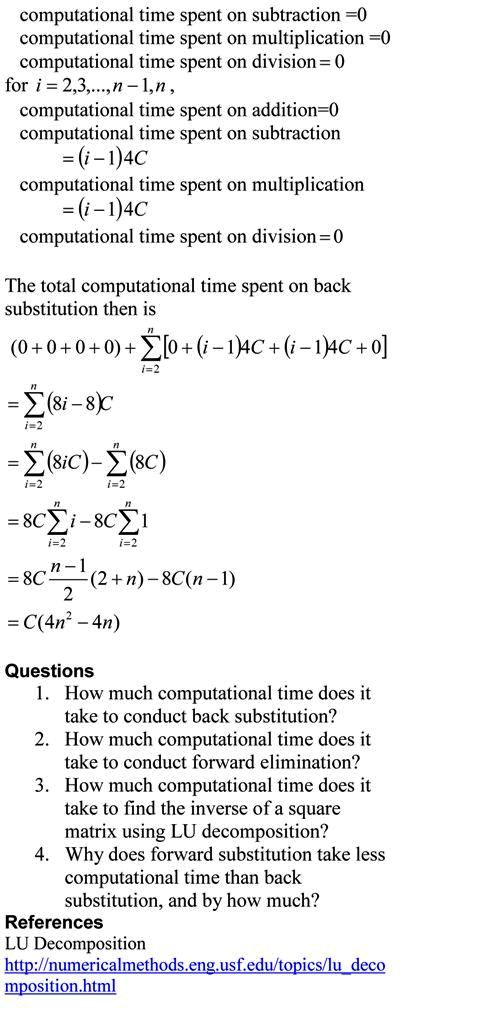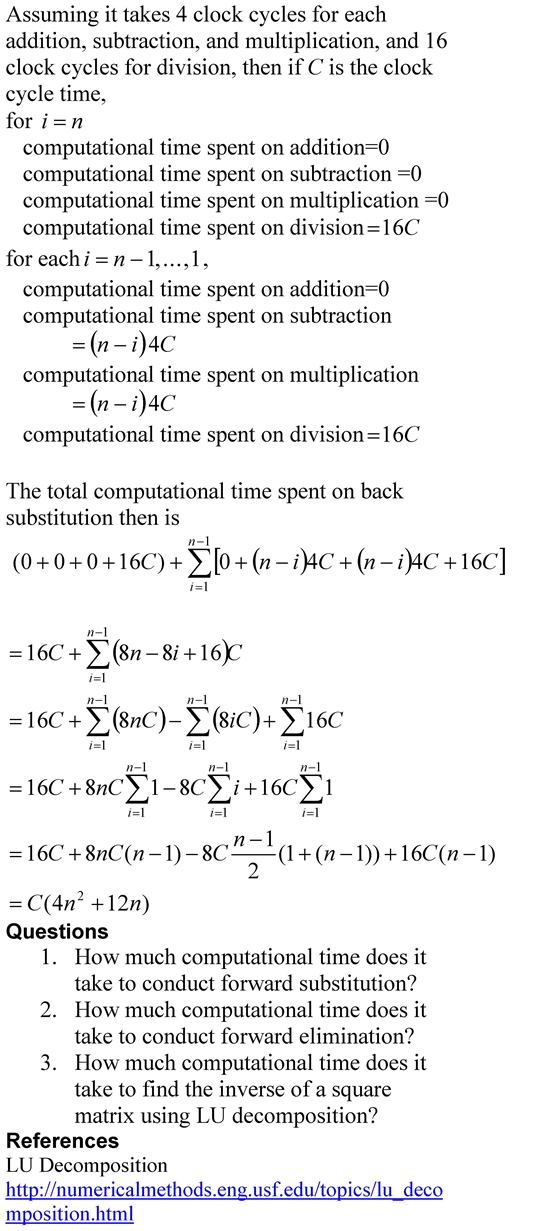## Matrix Algebra: LU Decomposition Method

Many university STEM major programs have reduced the credit hours for a course in Matrix Algebra or have simply dropped the course from their curriculum.   The content of Matrix Algebra in many cases is taught just in time where needed.  This approach can leave a student with many conceptual holes in the required knowledge of matrix algebra. In this series of blogs, we bring to you ten topics that are of immediate and intermediate interest for Matrix Algebra. Here is the seventh topic where we talk about solving a set of simultaneous linear equations using the LU decomposition method.  First, the LU decomposition method is discussed along with its motivation.  The LU decomposition method to find the inverse of a square matrix is discussed. Get all the resources in form of textbook content, lecture videos, multiple choice test, problem set, and PowerPoint presentation. LU Decomposition Method
This post is brought to you by
• Holistic Numerical Methods Open Course Ware:
• the textbooks on
• the Massive Open Online Course (MOOCs) available at

## How much computational time does it take to find the inverse of a square matrix using Gauss Jordan method?  Part 1 of 2.

Problem Statement

How much computational time does it take to find the inverse of a square matrix using Gauss Jordan method?  Part 1 of 2.

Solution

To understand the solution, you should be familiar with the Gauss Jordan method of finding the inverse of a square matrix.  Peter Young of UCSC describes it briefly in this pdf file while if you like watching an example via a video, you can see PatrickJMT doing so.  You also need to read a previous blog where we calculated the computational time needed for the forward elimination steps on a square matrix in the Naïve Gauss elimination method.   We are now ready to estimate the computational time required for Gauss Jordan method of finding the inverse of a square matrix.This post is brought to you by

• Holistic Numerical Methods Open Course Ware:
• the textbooks on
• the Massive Open Online Course (MOOCs) available at

## Computational Time for Forward Substitution

In the previous blog, we found the computatational time for back substitution. This is a blog that will show you how we can find the approximate time it takes to conduct forward substitution, while solving simultaneous linear equations. The blog assumes a AMD-K7 2.0GHz chip that uses 4 clock cycles for addition, subtraction and multiplication, while 16 clock cycles for division. Note that we are making reasonable approximations in this blog. Our main motto is to see what the computational time is proportional to – does the computational time double or quadruple if the number of equations is doubled.The pdf file of the solution is also available.

This post is brought to you by

## Computational Time for Back Substitution

This is a blog that will show you how we can find the approximate time it takes to conduct back substitution, while solving simultaneous linear equations using Gaussian elimination method. The blog assumes a AMD-K7 2.0GHz chip that uses 4 clock cycles for addition, subtraction and multiplication, and 16 clock cycles for division. Note that we are making reasonable approximations in this blog. Our main motto is to find how the computational time is related to the number of equations.The pdf file of the solution is also available.

This post is brought to you by

## LU Decomposition takes more computational time than Gaussian Elimination! What gives?

If you are solving a set of simultaneous linear equations, LU Decomposition method (involving forward elimination, forward substitution and back substitution) would use more computational time than Gaussian elimination (involving forward elimination and back substitution, but NO forward substitution).

So why use and waste time talking about LU Decomposition?

Because, LU Decomposition is computationally more efficient than Gaussian elimination when we are solving several sets of equations with the same coefficient matrix but different right hand sides. Case in point is when you are finding the inverse of a matrix [A]. If one is trying to find the inverse of nxn matrix, then it implies that one needs to solve n sets of simultaneous linear equations of [A][X]=[C] form with the n right hand sides [C] being the n columns of the nxn identity matrix, while the coefficient matrix [A] stays the same.

The computational time taken for solving a single set of n simultaneous linear equations is as follows:

• Forward elimination: Proportional to$\frac{n^3}{3}$
• Back substitution: Proportional to$\frac{n^2}{2}$
• Forward substitution: Proportional to$\frac{n^2}{2}$
• So for LU decomposition method used to find the inverse of a matrix, the computational time is proportional to$\frac{n^3}{3}+n( \frac{n^2}{2}+\frac{n^2}{2})=\frac{4n^3}{3}$. Remember that the forward elimination only needs to be done only once on [A] to generate the L and U matrices for the LU decomposition method. However the forward and back substitution need to be done n times.

Now for Gaussian Elimination used to find the inverse of a matrix, the computational time is proportional to$n \frac{n^3}{3} +n \frac{n^2}{2}=\frac{n^4}{3}+\frac{n^3}{2}$. Remember that both the forward elimination and back substitution need to be done n times.

Hence for large n, for LU Decomposition, the computational time is proportional to$\frac{4n^3}{3}$, while for Gaussian Elimination, the computational time is proportional to$\frac{n^4}{3}$. So for large n, the ratio of the computational time for Gaussian elimination to computational for LU Decomposition is${\frac{n^4}{3}}/{\frac{4n^3}{3}}=\frac{n}{4}$.

As an example, to find the inverse of a 2000×2000 coefficient matrix by Gaussian Elimination would take n/4=2000/4=500 times the time it would take to find the inverse by LU Decomposition.

So are you convinced now why we use LU Decomposition in certain cases? For textbook notes on this issue, examples of LU Decomposition to solve a set of equations, and finding inverse of a matrix using LU Decomposition, click here.

Reference: Numerical Methods for the STEM Undergraduate, http://numericalmethods.eng.usf.edu/topics/lu_decomposition.html

This post brought to you by Holistic Numerical Methods: Numerical Methods for the STEM undergraduate at http://numericalmethods.eng.usf.edu